Simulation of Vortex Ring Formation and the Effect of Piston VelocityProgram on the Formation Number | OMICS International
Fluid Mechanics: Open Access

# Simulation of Vortex Ring Formation and the Effect of Piston VelocityProgram on the Formation Number

Peng J*

Department of Mechanical Engineering, University of Alaska Anchorage, Anchorage, AK 99508, USA

*Corresponding Author:
Peng J
Department of Mechanical Engineering
Anchorage, AK, USA
Tel: +1 907-786-1800
E-mail: [email protected]

Received Date: September 01, 2015 Accepted Date: September 19, 2015 Published Date: September 28, 2015

Citation: Peng J (2015) Simulation of Vortex Ring Formation and the Effect of Piston Velocity Program on the Formation Number. J Vortex Sci Technol 2:120. doi:10.4172/2090-8369.1000120

Copyright: © 2015 Peng J. This is an open-access article distributed under the terms of the Creative Commons Attribution License, which permits unrestricted use, distribution, and reproduction in any medium, provided the original author and source are credited.

Visit for more related articles at Fluid Mechanics: Open Access

#### Abstract

Vortex ring formation is a limited growth process. Upon reaching a non-dimensional formation number, the vortex ring pinches off and secondary vortices form in the trailing jet. However, since a larger vortex ring is associated with higher propulsive efficiency, it is desired to delay vortex ring pinch-off. In this study, a numerical model based on vortex sheet method was used to study vortex ring formation from a piston-cylinder apparatus, with the focus on the vortex ring pinch-off at formation number F. Studies on the various piston velocity programs showed that piston acceleration is able to delay the vortex ring pinch-off and the formation of secondary vortices. The delay is more significant when the vortex ring pinch-off occurs while the piston is accelerating. Acceleration of piston before a constant velocity period would still delay the vortex ring pinch-off, but to a much smaller extent.

#### Keywords

Vortex ring, piston, formation, simulation

#### Introduction

Vortex rings, i.e., closed loops of vorticity, represent a unique form of vortices. The aspect of vortex ring dynamics most relevant to propulsion is its formation, when momentum is transferred into the fluid . Generation of vortex rings is believed to beneficial to propulsion. For example, it is found that force associated with vortex ring formation in a starting jet is greater than that of an equivalent steady jet, due to the over-pressure which is the increases in pressure at the nozzle exit to accelerate the otherwise still surrounding fluid in the formation process [2,3]. The contribution of overpressure makes the total ring impulse greater than that due to momentum flux alone during vortex ring formation. In addition, the benefit of vortex ring in propulsion also arises because of the entrainment of ambient fluid into the forming ring. The increase of wake vortex mass compared with a steady jet lowers the overall wake velocity and decreases the kinetic energy loss into the wake, thus increasing propulsive efficiency [4,5].

Generation of vortex ring is most commonly achieved by moving a piston through a cylinder tube submerged in a fluid, which has been used as the platform in many experimental and numerical studies. To exploit these benefits of vortex ring formation in propulsion, it is desired to generate larger, stronger rings. Using this piston-cylinder arrangement, Didden  first revealed that the main source of the vorticity in the forming ring is the vorticity flux from the ejected shear layer. The ring grows as the vorticity flux is entrained into it. However, there is a limit on the growth of a vortex ring from the jet shear layer. The ring formation process can be scaled by a non-dimensional formation time, defined as Tf=L/D=UT/D, where L is piston stroke distance, D the diameter of the circular jet, U the jet velocity, T the duration of the jet. For vortex ring from a starting jet in the classical piston-cylinder arrangement, the upper-limit for ring growth occurs at Tf ≈ 4  and this limit is called formation number F. The forming vortex ring accepts the vorticity flux from the jet shear layer until this limit is reached. Upon reaches this limit, vortex ring pinches off, and the trailing jet would not be entrained into the vortex ring and instead forms secondary vortices due to Kelvin-Helmholtz instability .

In some studies [1,7,8] the limiting vortex ring growth is explained by the Kelvin-Benjamin variational principle, which states that a steady-translating axis-touching vortex ring possesses maximum energy with respect to impulse-preserving iso-vortical perturbations.It follows from this principle that the pinch off occurs when the jet shear layer is no longer able to deliver energy at a rate compatible with the requirement of the Kelvin-Benjamin principle. In other words, at the formation number F, the non-dimensional energy of the vortex ring exceeds that of the jet, i.e., αjetring, where α=E/I1/2Γ3/2 is the nondimensional energy E normalized to impulse I and circulation Γ. In contrast, Linden and Turner  argue that it is the volume of fluid from the jet that limits vortex ring growth. Circulation, impulse and energy produced by the generator can be incorporated into the ring at considerably larger amount than the critical values obtained experimentally. Both theories predicted F ≈ 4.

The importance of the limit of vortex ring growth is that upon reaching this limit, the jet from the vortex generator stops contributing to the vortex ring and thus no long be able to utilize the benefits of vortex ring in propulsion. Therefore, it is believed that this limit represents the ‘optimal’ vortex ring formation in propulsion. Krueger and Gharib  compared the time-integrated force due to vortex rings formed at various Tf, and showed that the force production is maximized as the limit F is reached. Linden and Turner  also demonstrated that the formation of a single vortex ring at the formation number produces the maximum impulse for a given energy input, and, if ejected behind a swimming body, it produces the maximum thrust. By maximizing the size of the vortex rings formed during propulsion, the efficiency of momentum transport is also optimized .

Though F ≈ 4 for a starting vortex ring from a constant-velocity jet in a classical piston/cylinder arrangement, it is desired to increase F to generate larger vortex ring. Rosenfeld  used a numerical method to simulate vortex ring formation and showed that vortex pinch-off is relatively insensitive to trapezoid-shaped piston velocity program, though the velocity profile inside the cylinder would affect the formation number Shusser  used an analytical model to study the effect of time-dependent piston velocity programs on vortex ring formation. Their analytical model indicates that increasing the piston velocity increases the value of F and vice versa. Therefore, one would expect higher values of F for the decreasing nozzle exit diameter and lower values for the increasing nozzle exit diameter. This was demonstrated experimentally by Dabiri and Gharib  whose study proved that vortex ring pinch-off can be delayed with F up to 8 by reducing the size of nozzle exit during ring formation. The value of F is also affected by the background flow outside the jet. Dabiri and Gharib  demonstrated modest pinch-off delays of approximately 10% in F by immersing a piston-cylinder apparatus in a uniform bulk counterflow. The counter-flow could increase F by reducing the ring velocity and maintaining the ring proximity to the jet. In contrast, a co-flow in the same direction as the jet has the opposite effect, i.e., it reduces the vortex formation number .

Previous studies on the effect of the piston velocity program on vortex ring formation were mostly based on analytical models without the features of shear layer dynamics, or on empirical experiments with limited piston velocity programs. In this study, a numerical model was used to study the effect of the piston velocity program on vortex ring formation and its limit. The study would focus on the shear layer dynamics and its instability, which leads to the pinch off of the leading vortex ring and the formation of secondary vortices in the trailing jet.

#### Numerical Approach

In this study, the vortex ring formation dynamics was explored numerically with the purpose of identifying the effect of piston velocity program on vortex ring formation and its pinch-off. Nitsche and Krasny  used the axisymmetric vortex sheet model to simulate vortex ring formation in a piston-cylinder apparatus. They compared their simulation with the experiments of Didden  and proved that the model captures the basic features of the ring formation process. The method is based on Prandtl's boundary layer theory that the fluid motion can be decomposed into a viscous inner flow and an inviscid outer flow. It uses ideal fluid theory to model fluid motion in the outer flow, while the dissipation in the boundary layer is neglected. However, the features of flow separation and vortex shedding are incorporated into the model. It has been used to simulated vortex dynamics in a wide range of applications [13-19].

A similar vortex sheet model was used in this study (Figure 1). In the model, a bound vortex sheet is attached on solid boundary, e.g., the cylinder wall of the vortex generator. It is assumed that the flow only separates at the sharp edges (cylinder nozzle edges) and the only source of vorticity in the wake is a free vortex sheet that is shed from the edges. Due to the axisymmetric nature of the flow, both bound and free vortex sheets can be considered as consisting of circular vortex filaments centered on the axis of the cylinder. The instantaneous flow is considered as induced by both the bound and free vortex sheets.

Figure 1: Schematic of the vortex generator. Solid: Cylinder wall; arrows: uniform flow generated by the piston inside the cylinder.

The mathematical description of this numerical method is summarized as follows. The formulation is similar to Nitsche and Krasny  and interested readers can refer to it for detail. The stream function of the flow induced by a circular vortex filament with unit strength is expressed as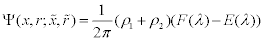(1)

Where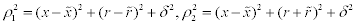and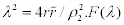and E(λ ) are the complete elliptic integrals of the first and second kind, and δ is the vortex-blob smoothing parameter, which removes the singularity in the non-regularized kernel . In the simulation, it is necessary to set δ >0 for filaments on the free vortex sheet for it to roll up smoothly, with δ=0 for filaments on the bound vortex sheet to prevent ill-conditioning in the equations to solve bound sheet strength . In this study, the value of the smoothing parameter is δ=0.2 for filaments on the free vortex sheet. As demonstrated in Nitsche and Krasny , the value of δ only affects the tightness of the spiral core, but not its location, the overall shape or the circulation of the vortex. The selected value for δ has been verified by a convergence analysis.

The velocity at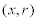induced by a circular filament at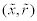with unit strength has axial and radial components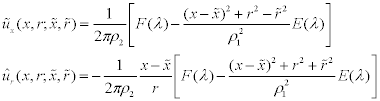(2)

For r=0, the velocity has the expression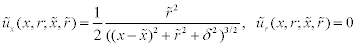(3)

Then the velocity of the flow at (x, r) is obtained by integrating along the bound and the free vortex sheet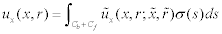,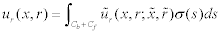(4)

Here σ(s) is the vortex sheet strength along the coordinate s. Cb is the contour of bound vortex sheet and Cf is the contour of free vortex sheet.

In practice, both bound and free vortex sheets are discretized by a set of circular filaments. The bound vortex sheet is represented as a fixed sets of filaments (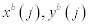) corresponding to the coordinates: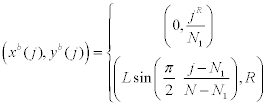(5)

where N1=11 and N=61 are the number of the vortex filaments on the cylinder inlet and the total number of the vortex filaments, respectively. The bound vortex filaments are shown in Figure 2. A time-stepping procedure is used to solve for the positions and strengths of these vortex filaments. At a given time t, the locations of bound vortex filaments are known since they are assigned and attached to the cylinder wall. The strength of each bound filament is solved by the no-penetration boundary condition, i.e., the component of flow velocity normal to the cylinder wall at any bound filament is zero. At a given time t, the strength of vortex filaments on the free sheet that were shed before t are known because they obey conservation of circulation and therefore each filament on the free vortex sheet carries the same amount of circulation at the time it was shed at edge of the cylinder. Once released into the wake, any filament on the free vortex sheet follows the flow velocity, and its position at time t can be obtained from its position and flow velocity up to time t. At each time step, a new vortex filament is released into the free sheet in the wake. The circulation shedding rate at the edge is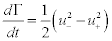(6)

where u+ and u- are the tangent slip velocity at the outer and inner surface of the cylinder edge, respectively. The new free vortex sheet filament has a circulation of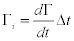, where Δt is the time step. The Euler’s method was used to calculate Γ.

Figure 2: Bound vortex filaments shown in circles. The vertical portion represents the inlet of the cylinder and the horizontal portion represents the cylinder wall.

On each time step of the calculation, one vortex filament is released into the free vortex sheet in the wake. The separation between consecutive filaments on the free vortex sheet may increase over time.To keep a smooth free vortex sheet, additional filaments are inserted into the free vortex sheet, which is a standard approach in the vortex sheet method [13-15]. In practice, additional filaments are inserted into the free sheet whenever the distance between consecutive filaments is larger than a critical value ε=0.2. A minimal number of filaments are inserted such that the distance between any two consecutive filaments on the refined free vortex sheet is smaller than ε. The strengths of the filaments inserted are determined by a piecewise cubic interpolation of the total circulation of all vortex filaments shed before time t, using the shedding time t as the interpolation parameter along the sheet.

The computation code used in this study was validated by comparing with some results in Nitsche and Krasny , shown in Figure 3. The axial and radial velocity components across the cylinder opening are simulated and are consistent with Nitsche and Krasny .

Figure 3: Validation of the numerical code by reproducing identical plots to those in Figure 8 of Nitsche and Krasny . (a) axial velocity u(L, r) across the cylinder opening; (b) axial velocity u(L, r) across the outside the cylinder at the opening; and (c) radial velocity v(L, r) at the opening. Numbers in the plots are simulation time in seconds. Note: Cylinder radius R=2.5 in this simulation to be consistent with Nitsche and Krasny.

In this study, the radius of the cylinder is R=2. The piston velocity program Up(t) consists a power-law acceleration followed by a constant velocity, described by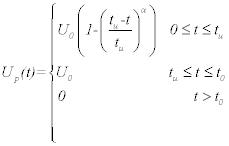(7)

Where tu is the acceleration period, t0 is the instance the piston stops, and U0=10 is the constant velocity after the acceleration. The parameter α is the power-law coefficient and the acceleration is linear when α=1. An example of the piston velocity program is shown in Figure 4. The non-dimensional formation time, is defined as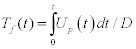(8)

#### Results

The study of Nitsche and Krasny  was limited to formation time smaller than its limiting value, which only generates a simple vortex ring without ring pinch-off. To demonstrate that the vortex sheet method is sufficient to capture the essential features of vortex formation and ring pinch-off, the numerical method was first used to compute the flow generated by a constant-velocity jet ejected from a piston-cylinder apparatus with Tf=6, large enough for the vortex ring pinch-off based on previous studies in which F ≈ 4. The vortex sheet from the vortex generator is shown in Figure 5. Clearly the jet shear layer is smooth at small Tf but as Tf increases, instability develops in the jet shear layer and secondary vortices are generated in the trailing jet. The computed flow is consistent with the empirical result of Gharib . The comparable features of vortex flow support the notion that the numerical method is appropriate for the analysis.

Figure 5: A vortex ring and the trailing jet from a piston-cylinder apparatus simulated by the vortex sheet method. The plots show the nozzle (black) and the vortex sheet (blue) at various Tf. The leading ring pinches off and a secondary vortex forms on the trailing jet when Tf>4. Only half of the symmetry plane is shown.

For a constant velocity jet, the formation number F=3.32. The value of the formation number F was determined in a similar way as in Gharib . The total circulation was calculated as a function of Tf. At vortex ring pinch-off, secondary vortices form in the trailing jet and are clearly separated from the leading ring. The circulation of the leading ring was then measured and its level intersect with the total circulation at the formation number F (Figure 6). In other words, when Tf reaches the formation number F, the vorticity and the circulation of the jet shear layer no longer contribute to the leading vortex ring, but rather go to the secondary vortices in the trailing jet. The value of the formation number is smaller than that determined in empirical experiments (F ≈ 4). One possible reason is that the numerical model does not include viscous dissipation. Therefore the instability in the jet shear layer is increased which would enhance secondary vortex ring formation in the trailing jet and separation from the leading ring.

Figure 6: Total circulation in the flow generated from a constantvelocity jet (solid) and the circulation of the leading ring after vortex pinch-off (dash). The two intersect at the formation number F.

Using the numerical model, vortex ring formation under various piston velocity programs was studied. First, piston velocity programs with tu=5, t0=5 and various power-law coefficients were used (Figure 7) because tu=t0, the piston is always in acceleration until it stops at t=t0 and there is no constant-velocity period. These piston velocity programs with acceleration only were designed to study the effect of the piston acceleration on vortex ring formation. The formation number F was determined for these piston velocity programs and the values are shown in Figure 8. For a linear acceleration piston velocity program (α=1), the formation number F=5.72 is significantly larger than F=3.32 for a constant-velocity program, indicating a rampingup piston velocity program is able to delay the formation of secondary vortices and to form a larger vortex ring. Power-law piston velocity programs are also able to delay the vortex ring pinch-off and to form larger rings but to a smaller extent compared with a linear acceleration program. And the formation number F decreases with an increasing power-law coefficient α. For α=5, the formation number F=3.62, just slightly larger than F=3.32 for a constant-velocity program. This wide range of F for various piston velocity programs can be explained by the acceleration rates of the piston velocity programs at the vortex ring pinch-off. Vortex pinch-off occurs after t=2 in all the piston velocity programs shown in Figure 7. The larger the power-law coefficient, the smaller the acceleration rate when pinch-off occurs. Furthermore, the larger the power-law coefficient, the more the piston approaches a constant velocity when pinch-off occurs. Therefore for piston velocity programs with large α, the effect of piston acceleration is smaller and the formation number approaches asymptotically to that of the constant-velocity program, as demonstrated in Figure 8.

Figure 7: Piston velocity program with acceleration only: tu=5, t0=5 with various power-law coefficientα in eqn.

Figure 8: Formation number F for piston velocity programs in Figure 6 with various power law coefficients.

Then piston velocity programs with a constant velocity period after the initial acceleration were studied. In these piston velocity programs, tu=2 and t0=4. The piston first accelerates from t=0 to t=tu and then moves at the constant velocity until it stops at t=t0. The same powerlaw coefficients were used for the acceleration phase (Figure 9). The formation number F was determined for these piston velocity programs and the values are shown in Figure 10. The formation number F=4.06 for α=1 and decreases to F=3.43 for α=5. The acceleration of the piston still delays the vortex ring pinch-off and the formation of secondary vortices, as the values of the formation number F are still larger than that of a constant-velocity program (F=3.32). The power law coefficient α also affects the formation number F in a similar way, as an increasing α reduces F. However, though the power law coefficients are the same as in Figure 8, the range of formation number F is not as big. The reason for this smaller range of formation number F is that vortex ring pinchoff occurs after t=2 when the acceleration of piston is complete, in all the piston velocity programs in Figure 9. Therefore at pinch-off, the pistons are not accelerating, but moving at the same constant speed. Acceleration before the constant velocity phase is still able to delay the vortex pinch-off and form larger rings, but the effect is not as significant as when the piston is still accelerating.

Figure 9: Piston velocity program with acceleration only: tu=2, t0=4 with various power-law coefficientα in eqn.

Figure 10: Formation number F for piston velocity programs in Figure 7 with various power law coefficients.

The piston velocity programs in Figure 9 are considered as a combination of piston acceleration and constant velocity. Therefore it is reasonable that the formation number F is between the value for the acceleration only program and a constant velocity program. For example, at linear acceleration (α=1), the formation number F=4.06 for piston program with the acceleration and constant velocity period, which is just slightly larger than that of a constant-velocity program (F=3.32) but significantly smaller than that in the acceleration only piston program (F=5.72). Because vortex ring pinch-off occurs during the constant velocity period, this implies that the piston acceleration rate at the vortex ring pinch-off is the dominant factor in delay in vortex ring pinch-off and formation of secondary vortices, even though the piston acceleration preceding the vortex pinch-off still has some similar effect, though to a lesser degree. As the power-law coefficient α increases, the piston acceleration rate at vortex ring pinch-off in the acceleration only piston programs decreases and approaches to constant velocity. Therefore, the difference between the acceleration only and acceleration with constant velocity programs becomes smaller. For example, in piston velocity programs with α=5, the formation number F=3.43 for the acceleration with constant velocity program, which is within a small region between F=3.32 for the constant velocity piston program and F=3.62 for the acceleration program.

#### Conclusion and Discussion

In this study, a numerical model based on vortex sheet method was used to study vortex ring formation from a piston-cylinder system, with the focus on the limited vortex ring growth at ring pinch-off at formation number F. Studies on the various piston velocity programs showed that piston acceleration is able to delay the vortex ring pinch-off and the formation of secondary vortices. The delay is more significant when the vortex ring pinch-off occurs while the piston is accelerating. Acceleration of piston before a constant velocity period would still delay the vortex ring pinch-off in the constant-velocity period, but to a much smaller extent.

The delay effect of the piston acceleration on vortex ring pinchoff can be explained by the comparison of vortex ring velocity and jet velocity. In previous studies, the limited growth of vortex ring can be explained by that the pinch off occurs when the jet shear layer is no longer able to deliver energy at a rate compatible with the requirement of the Kelvin-Benjamin principle . Or equivalently, vortex ring pinch-off occurs when the translational velocity of the ring is larger than that of the jet . This explains the delay of vortex ring pinch-off for an accelerating piston-velocity program. When the piston is acceleration, the jet velocity also accelerates. Therefore the trailing shear layer is less likely to fall behind the leading ring for the vortex ring pinch-off to occur. This is the reason that piston acceleration can help delay vortex ring pinchoff and the formation of secondary vortices in the trialing jet.

#### Acknowledgement

The author thanks the support from National Science Foundation grant CBET- 1449347.

#### References

Select your language of interest to view the total content in your interested language

### Article Usage

• Total views: 13676
• [From(publication date):
October-2015 - Mar 31, 2020]
• Breakdown by view type
• HTML page views : 9656Can't read the image? click here to refresh